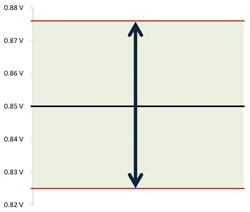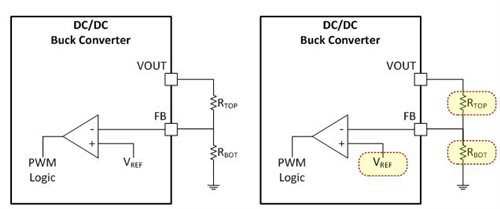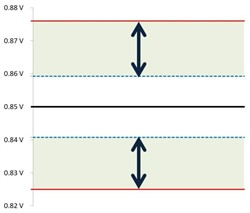# Powering FPGAs - What to consider with Xilinx UltraScale+ FPGAs

Other Parts Discussed in Post: TPS546C20A

Have you ever driven across a one-lane bridge or been in traffic with cars on either side? In such instances, there may only be a few feet of margin of error when steering. If your car was wider, the margin of error would decrease and steering would require more precision. The inverse is also true; the margin of error would increase if the car was narrower.

The rails on Xilinx UltraScale+ devices aren’t much different; there is an operating range in which the power supply’s output must remain, and the supply itself has a given “width” based on a deviation from the target output voltage. So how do you decrease this deviation from the target output voltage? The first thing to do is to identify factors that can impact output voltage accuracy. In this post, I will discuss the errors introduced by reference voltage accuracy and feedback resistor tolerance, and methods that reduce or eliminate these errors when choosing DC/DC buck converters for your UltraScale+ application.

To understand why this deviation from the target voltage is so important, let’s consider a common rail rating for UltraScale+ devices for a particular speed grade shown in Table 1. Please note that this rating could differ depending on your particular device’s speed grade. You should always consult the data sheet for your particular device.

 Min Typ Max Unit 0.825 0.850 0.876 V

Table 1: Common voltage ratings for VCCINT, VCCINT_IO, and VCCBRAM for Kintex, Virtex and Zync UltraScale+ devices

Now, let’s visualize what those numbers mean in terms of operating range. Figure 1 shows the full range of AC ripple allowed by the power supply’s output voltage.Figure 1: Power supply’s AC ripple output voltage range

According to Figure 1, there is approximately 50mV that the power supply’s output can deviate from the target voltage of 0.85V, or ±25mV (3% of the target voltage) for AC ripple. Actual load current and transient specifications, including transient step size and slew rate, would depend on resource usage of UltraScale+ devices, but you can estimate them using the Xilinx Power Estimator (XPE) tool.

Figure 2 shows a simplified feedback divider circuit for a DC/DC buck converter, highlighting those components that can decrease the output voltage accuracy. This is a generalization of the actual circuit; certain devices may have different naming conventions and use different pins altogether. The output voltage is divided down and sensed at the input of an error amplifier, where it is compared to a reference voltage (VREF). The output of the error amplifier (a magnitude of the difference between the two inputs) is used to set the pulse-width modulation (PWM) logic accordingly, in order to keep your output voltage to the desired target voltage. Altering the divider ratio or the value of VREF impacts the output voltage, so what happens when you factor in the reference voltage accuracy and feedback resistor tolerance? In order to answer this question, let’s work through an example based on a typical design.Figure 2: A simplified resistive feedback divider circuit (left); the same circuit with components that can decrease output voltage accuracy highlighted (right)

For this example, I’ll use resistors with a 1% tolerance rating for the feedback divider. The reference voltage will depend on the device, but let’s say that it that has a rated reference voltage of 0.6V with ±0.5% accuracy, as shown in Table 2. You can find this reference value and any deviation over temperature in a device’s data sheet.

 Parameter Min Typ Max Unit VREF 0.597 0.600 0.603 V

Table 2: Common reference voltage rating for TJ = 25°C

You now can calculate the error introduced by using:

• The resistor tolerance of the top resistor, TR_TOP, and the bottom resistor, TR_BOT, since they are equal: TR_TOP = TR_BOT = TR.
• The deviation in the reference voltage: ΔVREF.
• The typical reference voltage: VREF.
• The target output voltage: VOUT.

Plugging these terms into Equation 1:

Let’s revisit the initial graph showing the full range of AC ripple that the power supply’s output voltage allows, with the accuracy error just computed. Figure 3 shows three regions: The white region surrounding the target output voltage is the accuracy error for the previous example, and the two light-green regions are the remainder of allowable AC ripple. Originally this number was 50mV, or ±25mV. After you consider the accuracy error, the new value is approximately 32mV, or ±16mV. This is less than two-thirds the original operating range.Figure 3: Power supply AC ripple output voltage range for VREF = 0.6V and TR = 1%

So what can you do to reduce this output voltage accuracy error? Higher-precision feedback resistors could certainly work, especially with a rated tolerance of 0.1%, for instance. But what if you could eliminate the error introduced by the resistor tolerance completely for this rail, or in some cases reduce the reference voltage error to negligible values?

To eliminate the error introduced by the tolerance of the feedback resistors, you simply need to choose a device that has the option to adjust the reference voltage such that VREF = VOUT. If you look back over the equation used to calculate the output voltage accuracy error percentage, setting VREF = VOUT causes the first term to go to zero, leaving you with the deviation in the reference voltage. This also means that you should omit the bottom resistor and consult the data sheet when choosing the top resistor.

Reducing the deviation in the reference voltage requires choosing a device that allows trimming the reference voltage with a high resolution. Let’s look at several of these devices in Table 3 and bring it all together in Table 4.

Table 3 highlights a few key features, including the ability to set the reference voltage to 0.85V, the PMBus interface and the ability to trim the reference voltage with a high level of accuracy (VREF trim) via PMBus commands. Setting the reference voltage for these particular devices requires a single resistor from the VSEL pin to ground. Please see the corresponding data sheet for resistor values and recommendations.

 VREF range 0.6V to 1.1V 0.6V to 1.2V 0.6V to 1.2V 0.35V to 1.65V VREF resolution 50mV steps* 50mV steps 50mV steps 1.953mV steps** PMBus – – Yes Yes VREF trim – – – Yes Current rating 25A 25A 25A 35A – 70A*** Comments *0.65V is not a reference voltage option **A high-resolution setting requires the use of PMBus   ***Device can be paralleled to a second TPS546C20A

Table 3: DC/DC buck converters that result in minimal accuracy errors in UltraScale+ applications

Table 4 summarizes the output voltage accuracy error for several configurations. VREF was set equal to VOUT in calculating the accuracy error and allowable AC ripple for each DC/DC buck converter. Each of the calculations for the TPS546C20A device used a trimmed VREF.

 VOUT accuracy error Allowable AC ripple ± AC ripple allowed VREF = 0.6V TR_TOP = TR_BOT = 1% ±~1.088% 32mV 16mV VREF = 0.6V TR_TOP = TR_BOT = 0.1% ±~0.559% 42mV 21mV TPS543B20 ±0.5% 43mV 21.5mV TPS548B22 ±0.5% 43mV 21.5mV TPS549B22 ±0.5% 43mV 21.5mV TPS546C20A ±0.229% 49mV 24.5mV

Table 4: Output voltage accuracy error for several configurations

The functionality of several Texas Instruments devices resulted in a gain of over 0.5% output voltage accuracy when compared to a typical design. In cases where the reference voltage was trimmed on the TPS546C20A, the deviation from the ideal case was minimal. These accuracy gains resulted in a larger operating range for the device’s AC ripple, which is something to consider when designing in the UltraScale+ family.